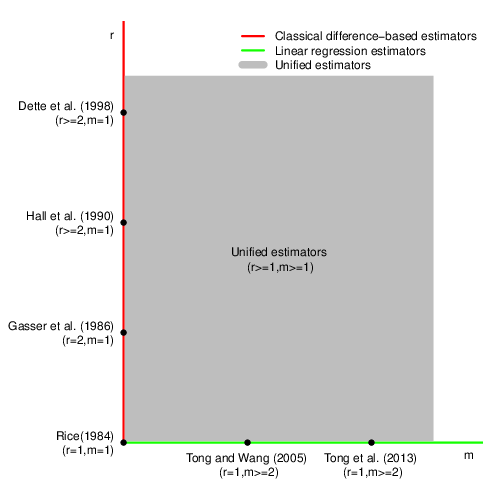Publications in nonparametric and semiparametric regression

-- * denotes students under my supervision.
-- The research in this area has been supported by the National Natural Science Foundation of China (NSFC) with Grant No. 11671338.

1. Sanying Feng, Gaorong Li, Heng Peng and Tiejun Tong (2020)
Varying coefficient panel data model with interactive fixed effects
Statistica Sinica, in press.

2. Shuwei Li, Tao Hu, Tiejun Tong and Jianguo Sun (2020)
Semi-parametric regression analysis of multivariate doubly-censored data
Statistical Modelling, in press.

3. Fengyang He, Huixia Judy Wang and Tiejun Tong (2020)
Extremal linear quantile regression with Weibull-type tails
Statistica Sinica, in press.

4. Sanying Feng, Gaorong Li, Tiejun Tong and Shuanghua Luo (2020)
Testing for heteroskedasticity in two-way fixed effects panel data models
Journal of Applied Statistics, 47: 91-116.

5. Wenwu Wang, Ping Yu, Lu Lin and Tiejun Tong (2019)
Robust estimation of derivatives using locally weighted least absolute deviation regression
Journal of Machine Learning Research, 20(60): 1−49.

6. Yiping Yang, Tiejun Tong and Gaorong Li (2019)
SIMEX estimation for single-index model with covariate measurement error
AStA Advances in Statistical Analysis, 103: 137-161.
-- The paper has been recognized as a highly cited paper in ISI Web of Science.

7. Yuejin Zhou, Yebin Cheng, Wenlin Dai* and Tiejun Tong (2018)
Optimal difference-based estimation for partially linear models
Computational Statistics, 33: 863-885.

8. Wenlin Dai*, Yuejin Zhou and Tiejun Tong (2018)
Testing discontinuities in nonparametric regression
Journal of Applied Statistics, 45: 450–473.

9. Wenlin Dai*, Tiejun Tong and Lixing Zhu (2017)
On the choice of difference sequence in a unified framework for variance estimation in nonparametric regression
Statistical Science, 32: 455-468.

10.-- As quoted from the abstract of the paper, the main contributions of this paper are as follows: "The unified framework has greatly enriched the existing literature on variance estimation that includes most existing estimators as special cases. More importantly, the unified framework has also provided a smart way to solve the challenging difference sequence selection problem that remains a long-standing controversial issue in nonparametric regression for several decades."

11. Wenlin Dai*, Tiejun Tong and Marc G. Genton (2016)
Optimal estimation of derivatives in nonparametric regression
Journal of Machine Learning Research, 17(164): 1-25.

12. Fengyang He, Yebin Cheng and Tiejun Tong (2016)
Estimation of high conditional quantiles using the Hill estimator of the tail index
Journal of Statistical Planning and Inference, 176: 64-77.

13. Fengyang He, Yebin Cheng and Tiejun Tong (2016)
Estimation of extreme conditional quantiles through an extrapolation of intermediate regression quantiles
Statistics & Probability Letters, 113: 30-37.

14. Yuejin Zhou, Yebin Cheng, Lie Wang and Tiejun Tong (2015)
Optimal difference-based variance estimation in heteroscedastic nonparametric regression
Statistica Sinica, 25: 1377-1397.

15. Wenlin Dai*, Yanyuan Ma, Tiejun Tong and Lixing Zhu (2015)
Difference-based variance estimation in nonparametric regression with repeated measurement data
Journal of Statistical Planning and Inference, 163: 1-20.

16. Yiping Yang, Gaorong Li and Tiejun Tong (2015)
Corrected empirical likelihood for a class of generalized linear measurement error models
Science China Mathematics, 58: 1523-1536.

17. Gaorong Li, Heng Peng, Kai Dong* and Tiejun Tong (2014)
Simultaneous confidence bands and hypothesis testing for single-index models
Statistica Sinica, 24: 937-955.

18. Wenlin Dai* and Tiejun Tong (2014)
Variance estimation in nonparametric regression with jump discontinuities
Journal of Applied Statistics, 41: 530-545.

19. Tiejun Tong, Yanyuan Ma and Yuedong Wang (2013)
Optimal variance estimation without estimating the mean function
Bernoulli, 19: 1839-1854.

20. Gaorong Li, Heng Peng and Tiejun Tong (2013)
Simultaneous confidence bands for nonparametric fixed effects panel data models
Economics Letters, 119: 229-232.

21. Tiejun Tong, Anna Liu and Yuedong Wang (2008)
Relative errors of difference-based variance estimators in nonparametric regression
Communications in Statistics - Theory and Methods, 37: 2890-2902.

22. Anna Liu, Tiejun Tong and Yuedong Wang (2007)
Smoothing spline estimation of variance function
Journal of Computational and Graphical Statistics, 16: 312-329.

23. Tiejun Tong and Yuedong Wang (2005)
Estimating residual variance in nonparametric regression using least squares
Biometrika, 92: 821-830.